# 机器学习模型可解释性实战-预测世界杯当场最佳

2019/08/15 07:02

### 构建模型

import numpy as np
import pandas as pd
import warnings
warnings.filterwarnings('ignore')

data.head()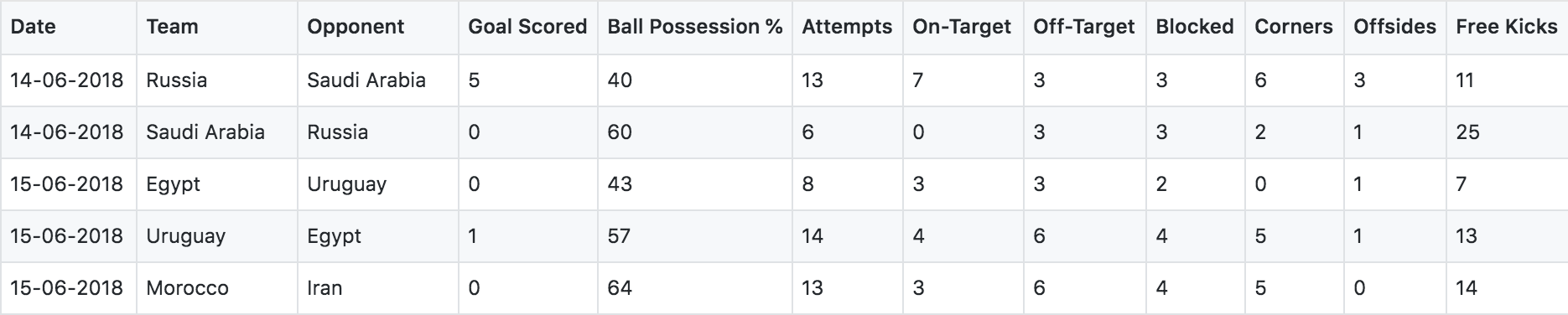from sklearn.model_selection import train_test_split
from sklearn.ensemble import RandomForestClassifier
from sklearn.tree import DecisionTreeClassifier

y = (data['Man of the Match'])
feature_names = [i for i in data.columns if data[i].dtype in [np.int64]]
X = data[feature_names]
train_X, val_X, train_y, val_y = train_test_split(X, y, random_state=1)
my_model_1 = RandomForestClassifier(random_state=0).fit(train_X, train_y)
my_model_2 = DecisionTreeClassifier(random_state=0).fit(train_X, train_y)

from sklearn import tree
import graphviz

tree_graph = tree.export_graphviz(my_model_2, out_file=None, feature_names=feature_names)
graphviz.Source(tree_graph)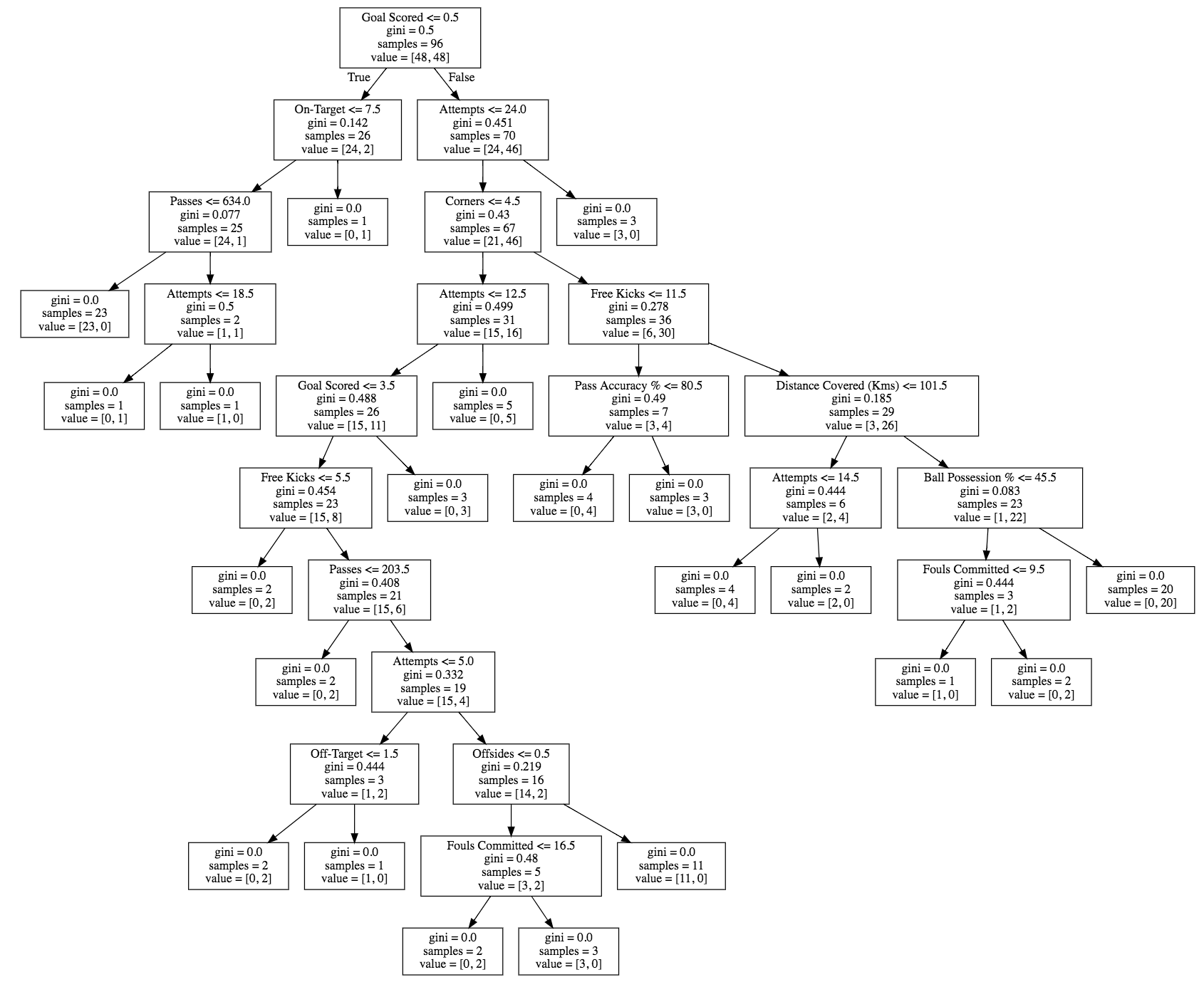### Permutation Importance

Permutation Importance提供了一个和模型无关的计算特征重要性的方法。Permutation的中文含义是排列的意思，该算法基本思路如下：

• 选择一个特征
• 在数据集上对该特征的所有值进行随机排列
• 计算新的预测结果
• 如果新旧结果的差异不大那么说明该特征重要性低，如果新旧结果差异显著，那么说明该特征对模型的影响也是比较显著的。

import eli5
from eli5.sklearn import PermutationImportance

perm = PermutationImportance(my_model_1, random_state=1).fit(val_X, val_y)
eli5.show_weights(perm, feature_names = val_X.columns.tolist())

### Partial Dependency Plot

PDP的基本思路就是控制其它所有特征不变，改变要分析的特征，看看它对预测结果的影响。ICE和PDP类似，ICE会显示所有实例上的分析结果。

from matplotlib import pyplot as plt
from pdpbox import pdp, get_dataset, info_plots

pdp_goals = pdp.pdp_isolate(model=my_model_1, dataset=val_X, model_features=feature_names, feature='Goal Scored')
pdp.pdp_plot(pdp_goals, 'Goal Scored', plot_pts_dist=True)
plt.show()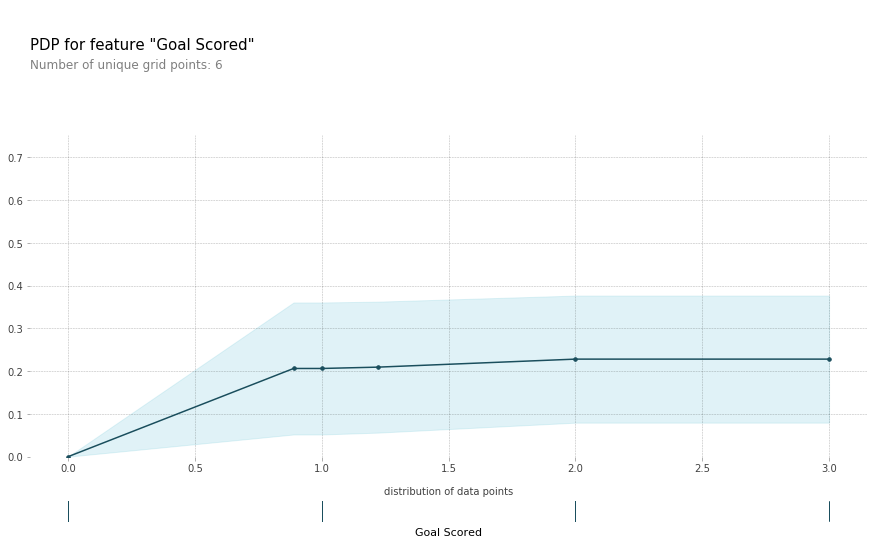feature_to_plot = 'Distance Covered (Kms)'
pdp_dist = pdp.pdp_isolate(model=my_model_1, dataset=val_X, model_features=feature_names, feature=feature_to_plot)

pdp.pdp_plot(pdp_dist, feature_to_plot, plot_pts_dist=True)
plt.show()

###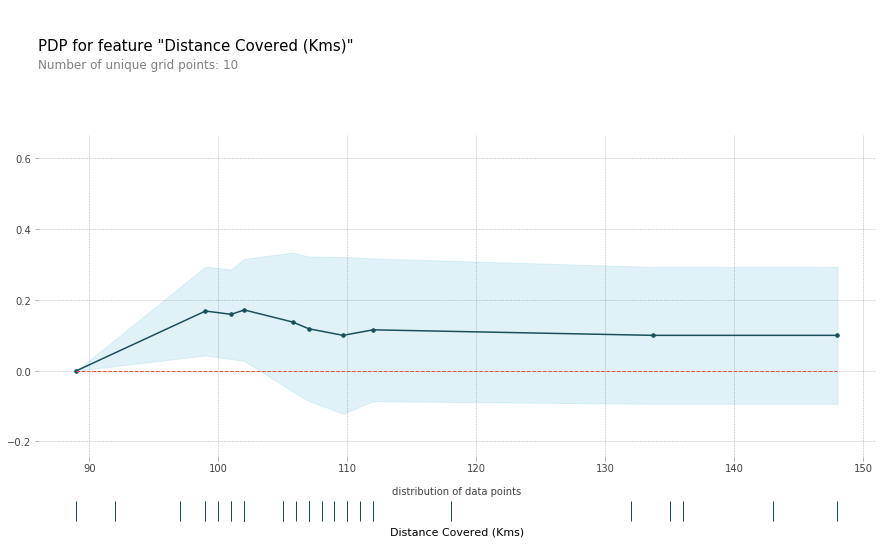Sklearn也提供了PDP的支持

import matplotlib.pyplot as plt
from sklearn.inspection import plot_partial_dependence
plot_partial_dependence(my_model_1, train_X,
['Goal Scored','Ball Possession %', 'Distance Covered (Kms)' , 'Corners', (0,1)],
feature_names, grid_resolution=50)
fig = plt.gcf()
fig.set_figheight(10)
fig.set_figwidth(10)

fig.suptitle('Partial dependence')
plt.subplots_adjust(top=0.9, bottom = 0.1, wspace = 0.8)  # tight_layout causes overlap with suptitle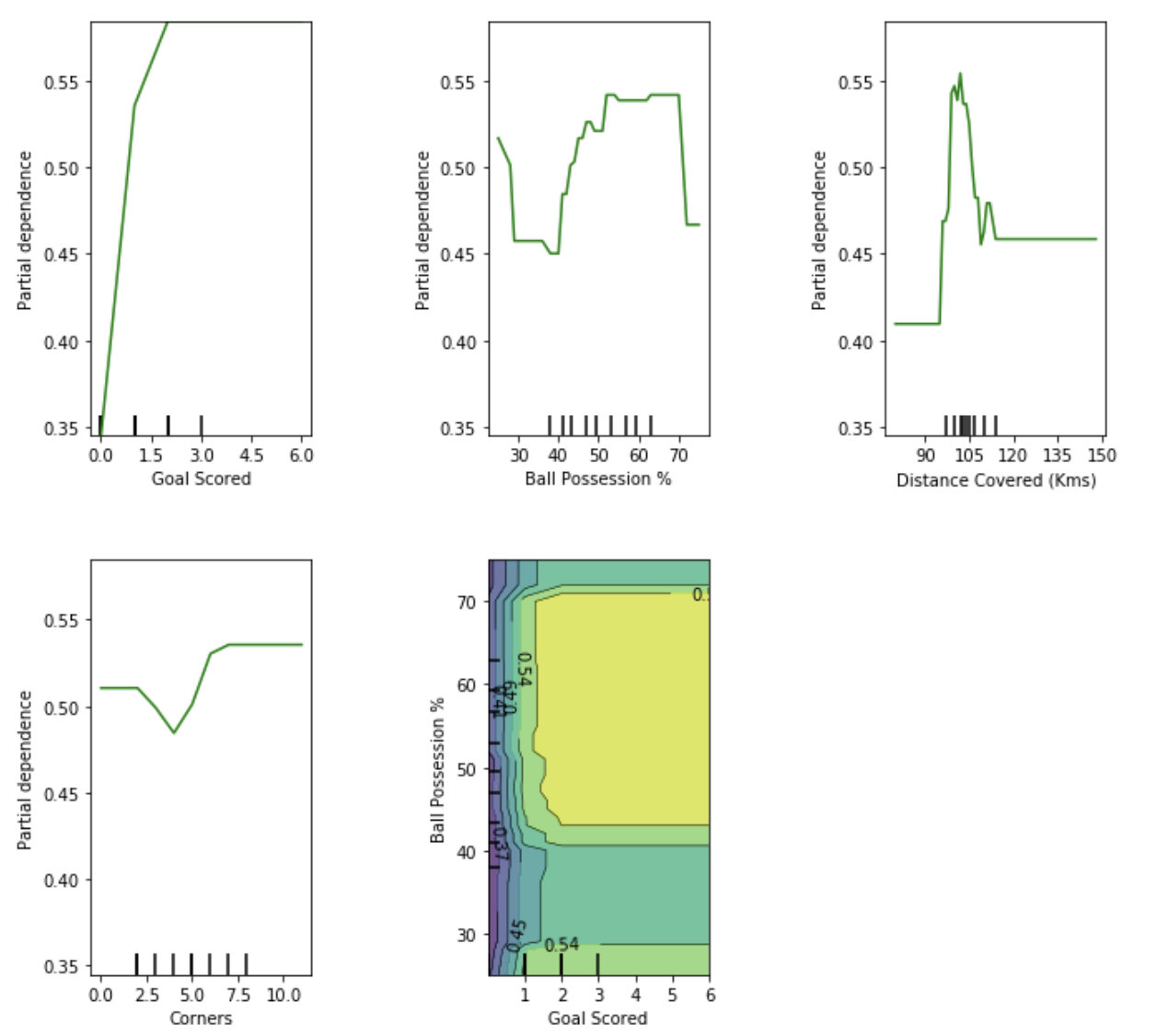PDP最多可以分析两个特征。上图中的最后一个PDP图就是包含了对进球数和控球两个特征的分析。

### Sharpley Value

PDP一般只针对某一个特征进行分析，最多两个，我们可以看出当分析两个特征的时候，PDP图已经不是一目了然的清楚了。Sharpley Value可以针对某一个数据实例，对所有的特征对预测的贡献作出分析。

Goal Scored                 2
Ball Possession %          38
Attempts                   13
On-Target                   7
Off-Target                  4
Blocked                     2
Corners                     6
Offsides                    1
Free Kicks                 18
Saves                       1
Pass Accuracy %            69
Passes                    399
Distance Covered (Kms)    148
Fouls Committed            25
Yellow Card                 1
Yellow & Red                0
Red                         0
Goals in PSO                3
Name: 118, dtype: int64
row_to_show = 5
data_for_prediction = val_X.iloc[row_to_show]  # use 1 row of data here. Could use multiple rows if desired
data_for_prediction_array = data_for_prediction.values.reshape(1, -1)

pred_1 = my_model_1.predict_proba(data_for_prediction_array)
pred_2 = my_model_2.predict_proba(data_for_prediction_array)
pred_1,pred_2
(array([[0.3, 0.7]]), array([[0., 1.]]))

import shap  # package used to calculate Shap values
explainer = shap.TreeExplainer(my_model_2)
shap_values = explainer.shap_values(data_for_prediction)
shap.initjs()
shap.force_plot(explainer.expected_value, shap_values, data_for_prediction)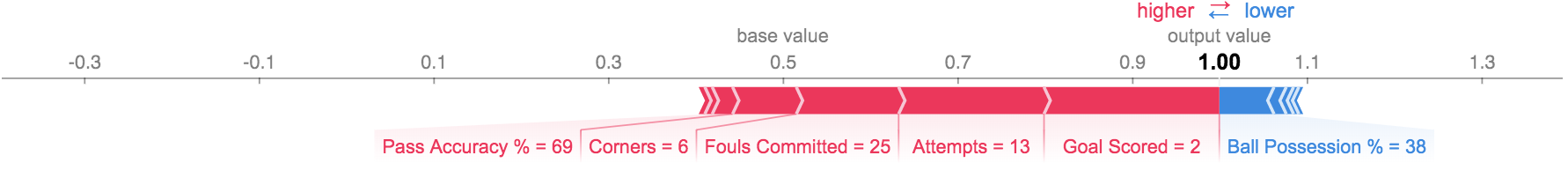KernelExplainer可以针对一般的模型进行Sharpley Value的分析，但是运算要慢一些。

k_explainer = shap.KernelExplainer(my_model_1.predict_proba, train_X)
k_shap_values = k_explainer.shap_values(data_for_prediction)
shap.initjs()
shap.force_plot(k_explainer.expected_value, k_shap_values, data_for_prediction)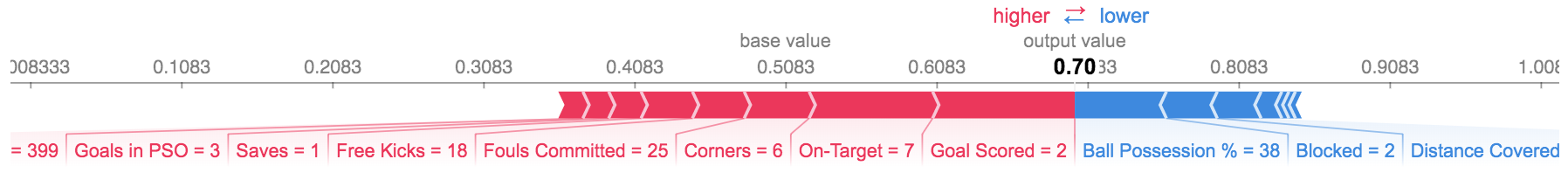Summary Plot给出了所有数据点的分析汇总

shap_values = k_explainer.shap_values(val_X)
shap.initjs()
shap.summary_plot(shap_values, val_X)

###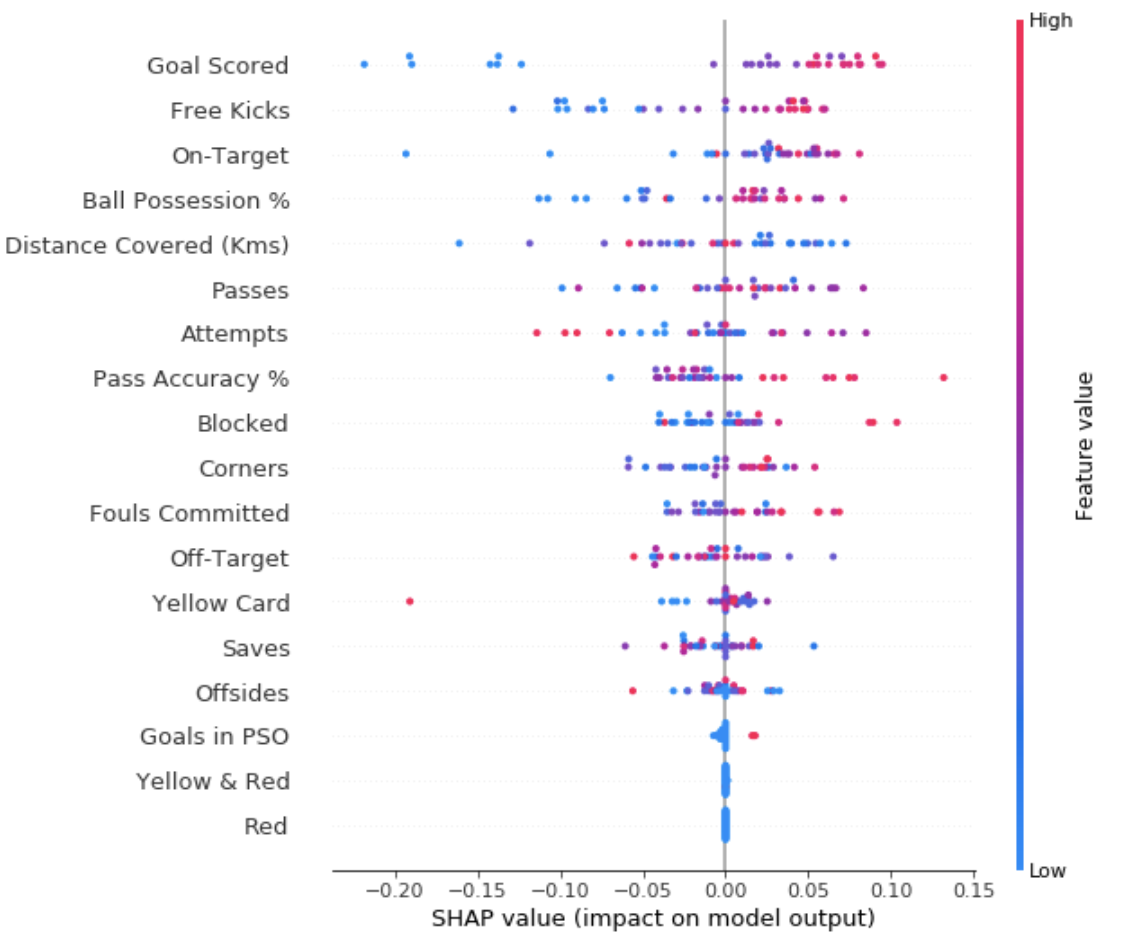explainer = shap.TreeExplainer(my_model_1)
# Calculate Shap values
shap_values = explainer.shap_values(X)
# make plot.
shap.dependence_plot('Ball Possession %', shap_values, X, interaction_index="Goal Scored")

### LIME

LIME 全称是 local interpretable model-agnostic explanations 直译是局部可解释的模型无关的解释，非常拗口。LIME针对某个实例，假定在局部，模型是简单的线性模型，对该数据点作出解释。import lime
import lime.lime_tabular
explainer = lime.lime_tabular.LimeTabularExplainer(train_X, feature_names=feature_names,
class_names=['No','Yes'],
discretize_continuous=False)

train_sample = train_X.sample(n=1)
pred_p_1 = my_model_1.predict_proba(train_sample.values)
pred_p_2 = my_model_2.predict_proba(train_sample.values)
pred_1 = my_model_1.predict(train_sample.values)
pred_2 = my_model_2.predict(train_sample.values)
pred_p_1,pred_1,pred_p_2,pred_2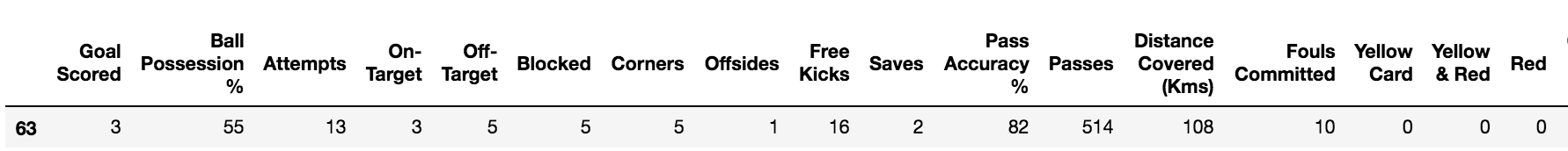exp = explainer.explain_instance(train_sample.values,
my_model_2.predict_proba,
num_features=len(feature_names),
top_labels=1)
exp.show_in_notebook(show_table=True, show_all=False)

### 参考### 作者的其它热门文章

0
1 收藏

0 评论
1 收藏
0